Question

If we take the third root of every term in a geometric sequence, is the resulting sequence also geometric? If so, what is its ratio? Justify your answer.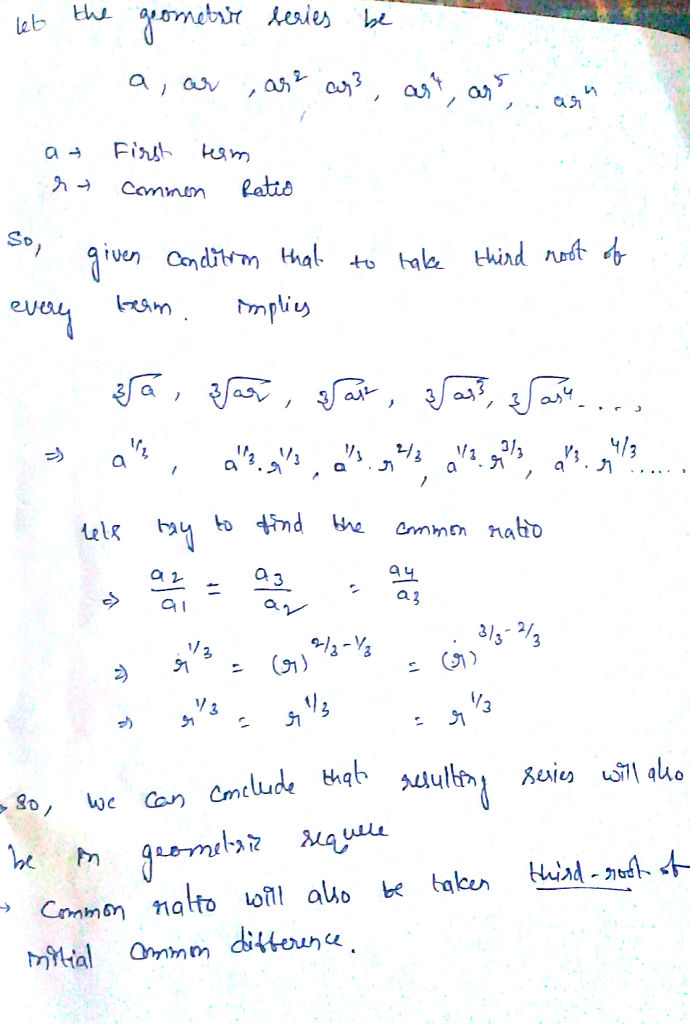#### Earn Coins

Coins can be redeemed for fabulous gifts.

Similar Homework Help Questions
• ### in a certain geometric sequence, the third term is 8 and the sixth term is 125

in a certain geometric sequence, the third term is 8 and the sixth term is 125. what is the first term?

• ### Given a geometric sequence whose third term 3/4 and seventh term is 243/64. Find the fifth...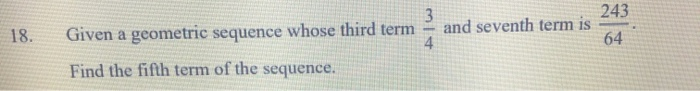Given a geometric sequence whose third term 3/4 and seventh term is 243/64. Find the fifth term of the sequence 3 243 18. and seventh term is Given a geometric sequence whose third term 4 64 Find the fifth term of the sequence.

• ### Find the sum of the first 28 terms of a geometric sequence whose third term is...

Find the sum of the first 28 terms of a geometric sequence whose third term is a3 = 6 and whose eighth term is a8 = 15.62.

• ### A sequence is formed by adding together the corresponding terms of a geometric sequence and and an arithmetic sequence

A sequence is formed by adding together the corresponding terms of a geometric sequence and and an arithmetic sequence.The common ratio of the geometric sequence is 2 and the common difference of the arithmetic sequence is 2.The first term of the new sequence is 1 and the second term is 7.Could you please help me find the third term of the new sequence.Thank you.

• ### 33. Find the fifth term and the nth term of the geometric sequence whose initial term...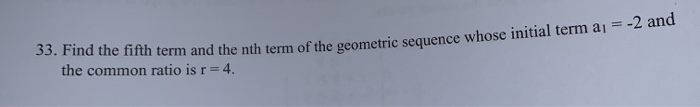33. Find the fifth term and the nth term of the geometric sequence whose initial term a1 = -2 and the common ratio is r=4.

• ### Determine the common ratio r, the fifth term, and the nth term of the geometric sequence....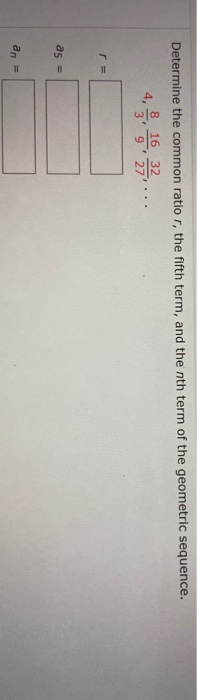Determine the common ratio r, the fifth term, and the nth term of the geometric sequence. 8 16 32 4, 3' 9' 271 as = an =

• ### find the 25th term of a geometric sequence having a common ratio r=1/4 and term a=3

find the 25th term of a geometric sequence having a common ratio r=1/4 and term a=3

• ### Determine 3rd term in the geometric sequence whose first term is -8 and whose common ratio...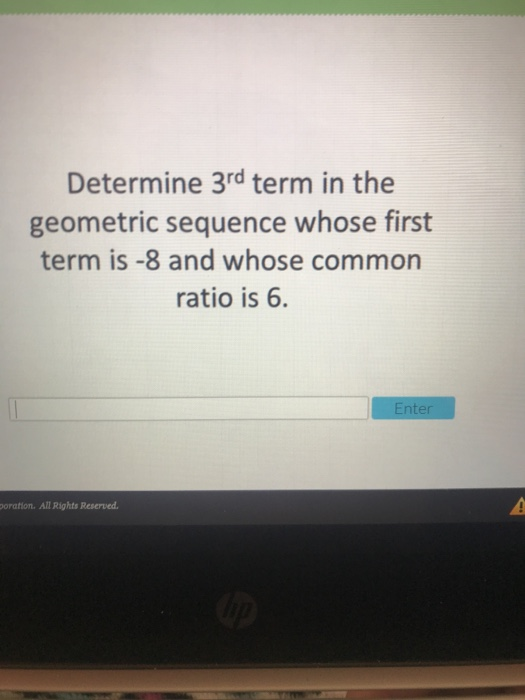Determine 3rd term in the geometric sequence whose first term is -8 and whose common ratio is 6 nter poration. All Rights Reserved

• ### Find the 6th term of the geometric sequence whose common ratio is 1/3 and whose first term is 7

Find the 6th term of the geometric sequence whose common ratio is 1/3 and whose first term is 7.